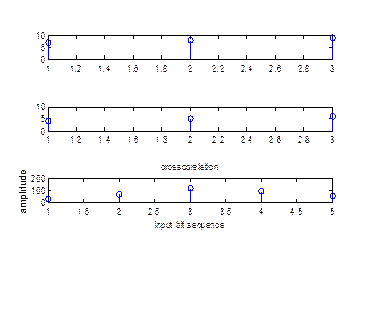EXPERIMENT NO.3

TITLE: COMPUTE AUTO CORRELATION AND CROSS CORRELATION OF TWO SEQUENCES

THEORY:-

Auto-correlation:-

Correlation is a mathematical tool used frequently in signal processing for analyzing function or series of values such as time domain signals correlation is mutual relationship between two or more random variables. Auto-correlation is correlation of signal with itself. This is unlike of two different signals.

Auto-correlation is useful for finding repeating patterns in a signal such as determining presence of periodic signal which has been buried under noise or identifying fundamental frequency of signal which doesnâ€™t actually contains that frequency components but implies it with many harmonic frequency.

Different definitions of auto-correlation are in us depending on field of study which is being considered and not all of them are equivalent. In some fields the term is used interchangeability with auto covariance.

In signal processing given signal f(t), the continues auto-correlation Rf(t) is the continues cross-correlation of f(t) with itself.

The auto-correlation of a periodic function is itself periodic with the very same period.

The auto-correlation of sum of two completely uncorrelated functions is the sum of auto-correlation each function separately.

Since auto-correlation is specific type of cross-correlation, it maintains all properties of cross-correlation.

The auto-correlation of a white noise signal will have strong peak at t=0 & will be close for all other T. This shows that a sampled instance of white noise signal is not statically correlated to sampled instance of same white noise signal at another time.

The Wiener-khinchin theorem relates the auto-correlation function to power spectral density via Fourier transform.

Cross-correlation:-

In signal processing cross-correlation is a measure of similarities of two waveforms as function of time lag applied to one of them. This is also known as a sliding dot product or inner product. It is commonly used for searching a long duration signal for a shorter known feature. It also has applications in pattern recognition, single practical analysis & neurophysiology.

The cross-correlation is similar in nature to convolution of two functions. Whereas convolution involves reversing signal & then shifting it & multiplying by another signal, correlation only involves shifting it & multiplying (no reversing).

In an auto-correlation, which is the cross-correlation of signal with itself, there will always be a peak at lag of zero.

If x & y are two independent random variables with probability distribution f & q respectively, then probability distribution of difference y - x is given by cross-correlation f * q . In contrast the convolution f *q gives probability distribution of the sum x + y.

In probability theorems & statistics the term cross-correlation us also sometimes used to referred to the convenience conv(x,y) between two random variables x & y in order to distinguish that concept from â€œCovarianceâ€ of a random variable x, which is understood to be matrix of covariance between the scalar components of x.

Properties:-

The cross-correlation of function f(t) & g(t) is equivalent to the convolution of f*(-t) & g(t)

i.e.f*g=(t ->f*(-t))*g

If either for g is hermitical then:

F * g= f * g

(f * g) * (f * g) = (f * f) * (g * g)

Analysis to convolution theorem

The cross-correlation satisfies,

F{ f * g} = ( F {f} )* F{g} ,

Where F denotes Fourier transform & an asterisk again indicates complex conjugates coupled with fast Fourier transform algorithms, this property is often explained for the efficiency numerical computation of cross-correlation.

The cross-correlation is related to the spectral density.

The cross-correlation of a convolution of f & h with function g is convolution of the correlation of f & g with kernel h:

(f * h) * g = h -> * (f * g)

MATLAB CODE

Auto and Cross Correlation

disp('autocorrelation and crosscorelation of sequences');

disp('4 autocorrelation 5 crosscorelation');

choice=input('enter the choice of corelation=');

switch(choice)

case{4}

x=input('enter the input seqence for auto,x=');

y=fliplr(x);

r=conv(x,y);

subplot(3,1,1);

stem(x);

subplot(3,1,2);

stem(y);

subplot(3,1,3);

stem(r);

xlabel('input bit sequence');

ylabel('amplitude');

title('autocorrelation');

case{5}

d=input('enter input sequence for cross,d=');

y=input('enter input sequence for cross,y=');

z=conv(d,y);

subplot(4,1,1);

stem(d);

subplot(4,1,2);

stem(y);

subplot(4,1,3);

stem(z);

xlabel('input bit sequence');

ylabel('amplitude');

title('crosscorelation');

end;

OUTPUT:-

autocorrelation and crosscorelation of sequences

4 autocorrelation 5 crosscorelation

enter the choice of corelation=5

enter input sequence for cross,d=[7 8 9]

enter input sequence for cross,y=[4 5 6]CONCLUSION